# How to Highlight Cells Containing Formulas in Excel

This post will guide you how to highlight cells in which contain formulas using Conditional Formatting feature in Excel. How do I conditionally format a cell if it contains formula using a User defined function in combination with Conditional Formatting feature in Excel 2013/2016.

Assuming that you have a list of data in range A1:C5, in which contain product names, sales values and formulas. And you want to only highlight cells if containing formula. How to accomplish it. This post will show you two methods to conditionally format cells if the cells contain formula.

## Method1: Highlight Cells Containing Formulas Using Defined Name

To highlight Cells containing formulas in your worksheet, you need to create a new range name IsFormula to your workbook. Just do the following steps:

Step1: go to Formulas tab, and click Name Manager button under Defined Names group. and the Name Manager dialog will open.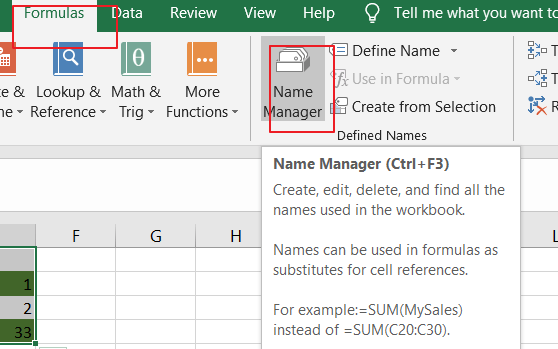Step2: click New button in the Name Manager dialog box, and the New Name dialog will open.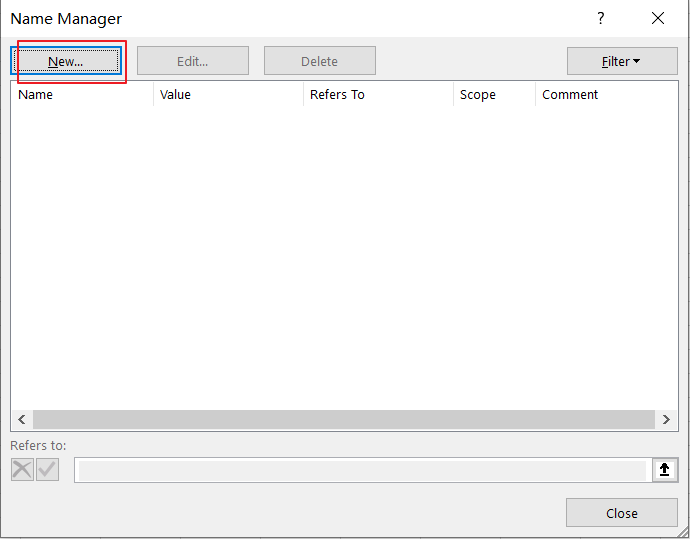Step3: type a name called IsFormula into the Name text box, and choose Workbook from the drop-down list of Scope. then you need to type the following formula into the Refers to text box. click Ok button.

=GET.CELL(48,INDIRECT(“rc”,FALSE))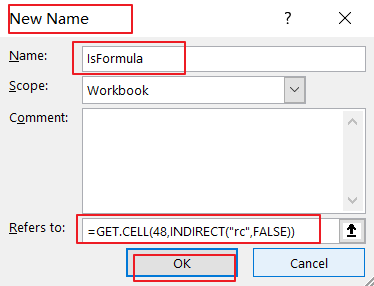Step4: select the range of cells on your worksheet to be conditioanlly formatted for formulas.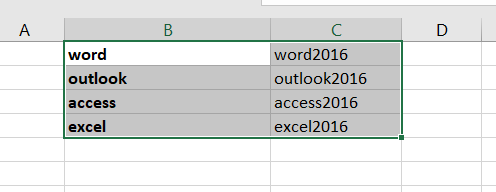Step5: go to Home tab, click Conditional Formatting command under Styles group, and select New Rule from the drop down menu list. And the New Formatting Rule dialog will open.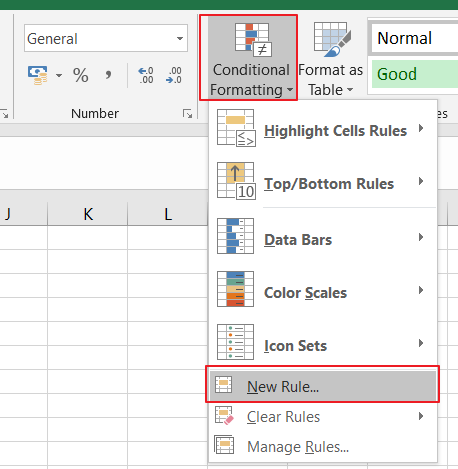Step6: click Use a formula to determine which cells to format option in the Select a Rule Type section in the New Formatting Rule dialog box, and type the following formula into the Format values where this formula is true text box.

=IsFormula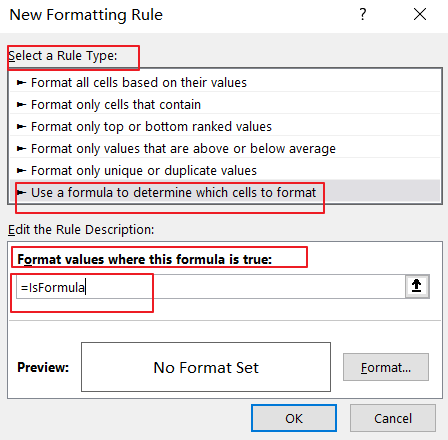Step7: click Format button, and the Format Cells dialog will open. swith to Fill tab in the Format Cells dialog box, and select one backgroud color as you need to highlight the cells with formulas. click Ok button to back to New Formatting Rule dialog box.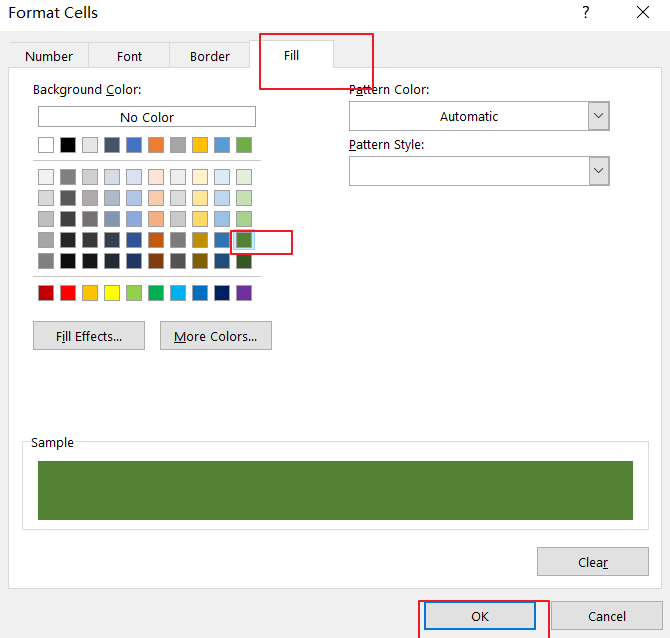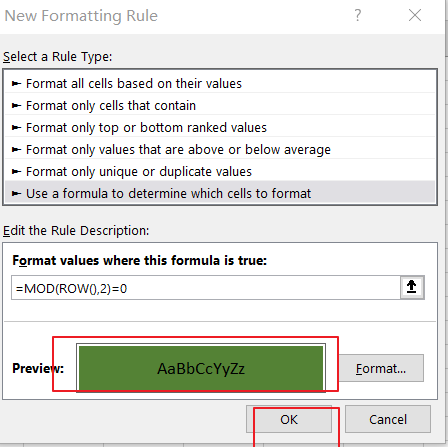Step8: click OK button, let’ see the last result: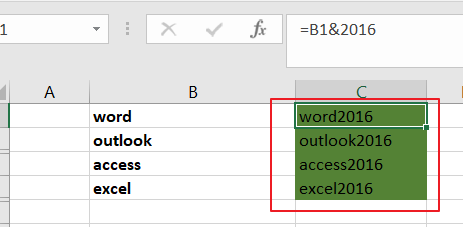## Method2: Highlight Cells Containing Formulas Using User Defined Function

You can also use an Excel User Defined Function in conbination with Condtional Formatting feature to highlight cells containing formuals. just do the following steps:

Step1# open your excel workbook and then click on “Visual Basic” command under DEVELOPER Tab, or just press “ALT+F11” shortcut.Step2#  then the “Visual Basic Editor” window will appear.

Step3# click “Insert” ->”Module” to create a new module.Step4# paste the below VBA code into the code window. Then clicking “Save” button.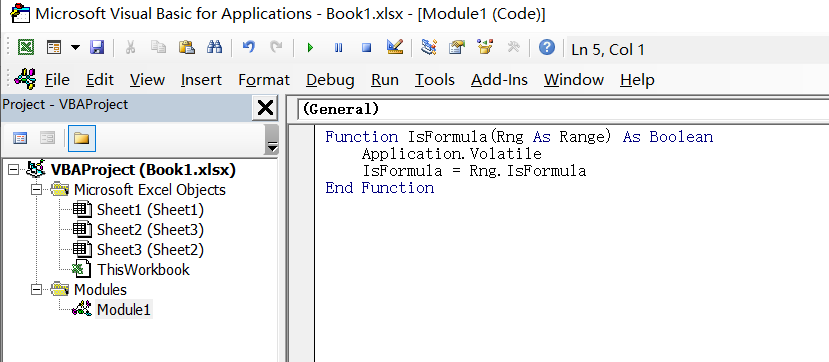```Function IsFormula(Rng As Range) As Boolean
Application.Volatile
IsFormula = Rng.IsFormula
End Function```

Then you need to repeat the above Step4-Step8 to highlight cells containing formulas in your selected range of cells.

Related Posts

How to Extract First Letter from Each Word in a Cell in Excel

This post will guide you how to extract first letter from each word in a given cell in Excel. How do I extract the first letter of each word in a range of names in your current worksheet in Microsoft ...

How to ignore Blank Cells while Concatenating cells in Excel

This post will guide you how to concatenate cells but ignore all blank cells in your worksheet in Excel. How do I concatenate cells but ignore blank cells with a formula in Excel. How to create a concatenate formula to ...

How to Calculate the Average Excluding the Smallest & Highest Numbers in Excel

Calculating the average for a batch of data is frequently used in our daily life. But for some cases like statistic the average score in a competition, or price analysis, we often calculate the average excluding the smallest and highest ...

How to Get the Maximum or Minimum Absolute Value in Excel

It is easy to find the maximum or minimum value in a batch of data in excel, but if this batch of numbers contains both positive and negative numbers, the maximum or minimum absolute value cannot be found out by ...

How to Count Only Numbers in Bold in a Range of Cells in Excel

This post will guide you how to Count only numbers with bold style in a range of cells in Excel. How do I Count on cells with bold font within a range of cells using User defined function in Excel ...

How to Sum Only Numbers in Bold in a Range of Cells in Excel

This post will guide you how to sum only numbers with bold style in a range of cells in Excel. How do I sum on cells with bold font within a range of cells using User defined function in Excel ...

How to Filter Formula in Excel

This post will guide you how to filter your data with an Formula in Excel. How do I filter cells with formulas through a User defined function in Excel 2013/2016. Filter Formula Assuming that you have a list of data ...

How to Count Cells by Font Color in Excel

This post will guide you how to count cells by font color in Excel. How do I Count the number of cells with specific cell Font color by using a User Defined function in Excel. Count Cells by Font Color ...

How to Extract Number from Text String in Excel

This post will guide you how to extract number from a given test string in Excel. How do I extract all numbers from string using a formula in Excel. How to get all number from a given test string using ...

How to Filter Data with Strikethrough Format in Excel

This post will guide you how to filter or delete rows with strikethrough format in your selected range of cells in Excel. How do I Filter by strikethrough formatted text with Find and Replace function in Excel 2013/2016. Or how ...

Sidebar